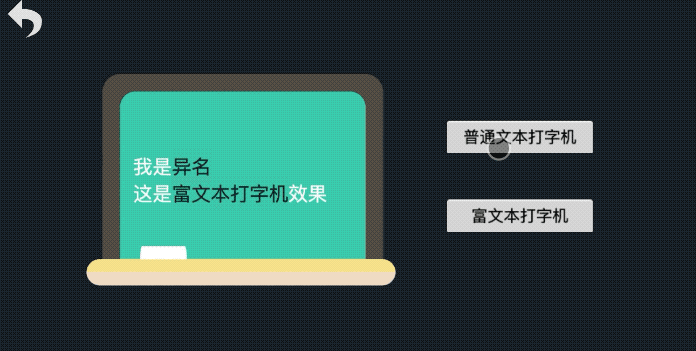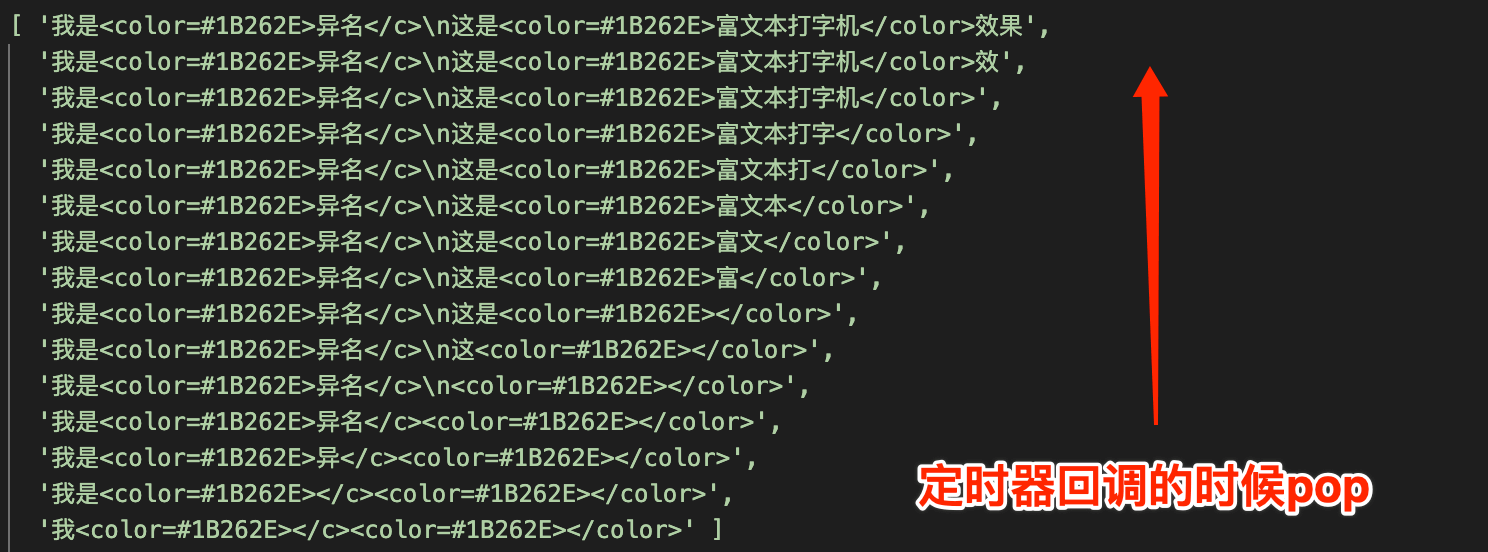# 效果演示# 实现思路

``````typerTimer: number = null; // 计时器Id
makeLaberTyper(str: string) {
let charArr = str.split('');
let charIdx = 0;

this.typerTimer && clearInterval(this.typerTimer);
this.typerTimer = setInterval(() => {
if (charIdx >= charArr.length) {
this.typerTimer && clearInterval(this.typerTimer);
} else {
charIdx += 1;
this.label.string = charArr.slice(0, charIdx).join('');
}
}, 50);
}
``````

`我是<color=#1B262E>异名</c>\n这是<color=#1B262E>富文本打字机</color>效果```````let str = '我是<color=#1B262E>异名</c>\n这是<color=#1B262E>富文本打字机</color>效果';
let charArr = str.replace(/<.+?\/?>/g, '').split('');
let tempStrArr = [str];

for (let i = charArr.length; i > 1; i--) {
let curStr = tempStrArr[charArr.length - i];
let lastIdx = curStr.lastIndexOf(charArr[i - 1]);
let prevStr = curStr.slice(0, lastIdx);
let nextStr = curStr.slice(lastIdx + 1, curStr.length);

tempStrArr.push(prevStr + nextStr);
}
console.log(tempStrArr)
``````# 效果预览2 个评论